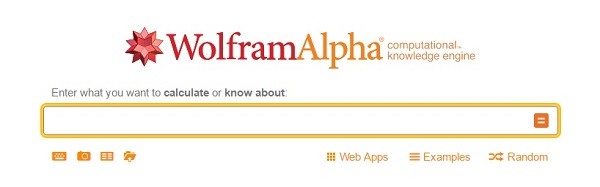-->

# Wolfram Alpha - A Computational Knowledge Engine

Posted by Sharath
Here introducing another way of computing various functionalities right from many sections of maths like calculas,trignometry, algebra etc, and another fields. So here is the application - Wolfram|Alpha. Wolfram|Alpha introduces a fundamentally new way to get knowledge and answers— not by searching the web, but by doing dynamic computations based on a vast collection of built-in data, algorithms, and methods. (extracted from the aboutus)Here almost 30+ webapps are there you to try out and it gives not only result but it gives more analysis or algorithmic way of explanations, and so many. Look out these examples and explore this website for your calculations.

Webapps:

Example 1:

Example 2:

So explore this amazing website and leave us a comment here how you liked it. For more such interesting websites check out our other posts